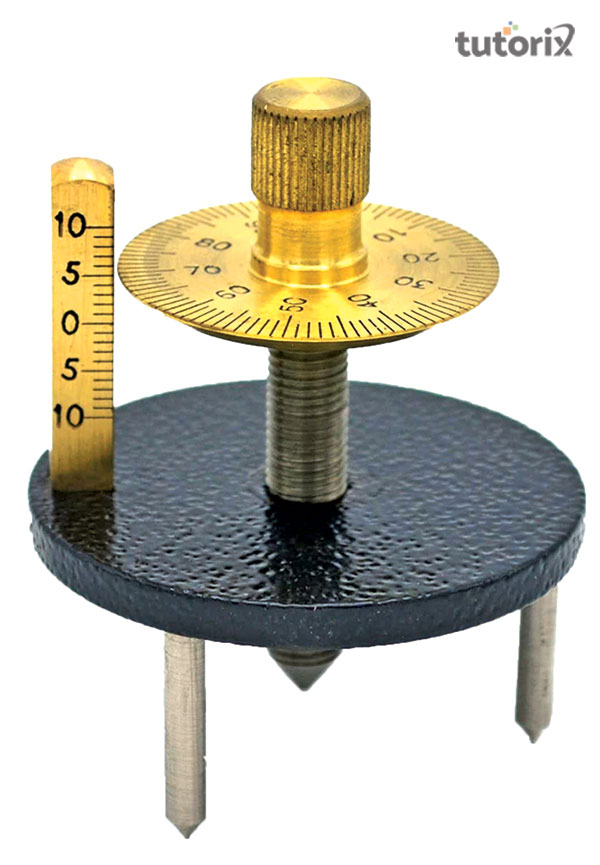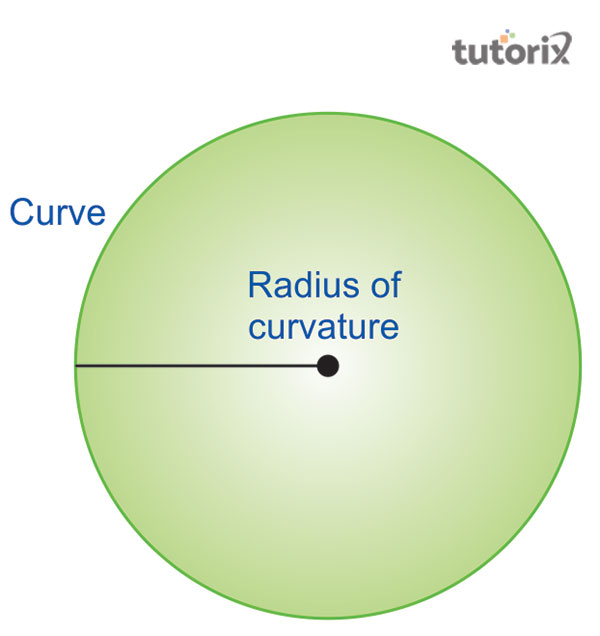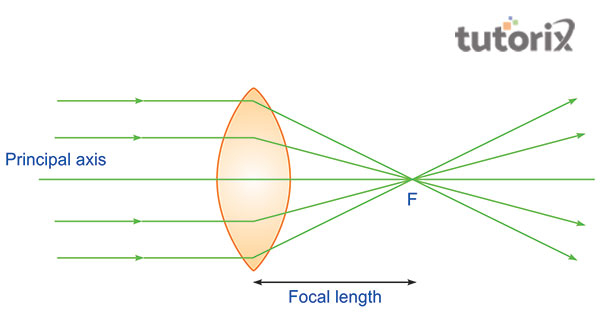# To determine radius of curvature of a given spherical surface by a Spherometer

Radius is the particular moving point of a normal circle which alters the position by itself with the moving curve. The curvature’s radius is the curve that makes the positioning depending on the curve location. The radius of curvature is the formation of the curved part of a particular lens where the radius is formed by the movement of the circle. In this tutorial, the calculation of the radius of curvature is highlighted by using a Spherometer which helps measure the triangular frame.

## Description of Spherometer

An instrument that helps to measure the three legs which are making the triangle with the formula is the ultimate process of estimating the radius of curvature. The spikes of the three portions create an equilateral triangle and lie radius of the circle.

The most exciting feature of a Spherometer is, that the central portion can move in the perpendicular direction that can help in the appropriate calculation of the radius of curvature. A desk in a circular shape is fixed on the head and includes a scale that marks the outer portion of the edges. All the different portions (legs of the Spherometer) are adjustable and the height is readable (Definitions, 2022). Additionally, one turn performed by a Spherometer is equivalent to 1mn.Figure 1: A Spherometer

## The Radius of Curvature FormulaThe radius of curvature of the curve is the radius of a circle at a particular position given. The movement of a circle can make the transformation in the curvature due to the changes brought in the moving circle’s radius (Lodder, 2018).

The character ‘R’ represents the formula to estimate the value of the radius of curvature. The curve derivates amount is flat to a curve and it can make a curve line at back with the scalar quantity (Sciencing, 2022).

Additionally, the curvature’s radius is an imaginary circle instead of the actual shape or image. The radius of curvature is the length from the vertex to the centre of curvature. ‘Y’ or the curve is equal to the f(x), where, ‘x’ represents the radius.

## Principle Focus and Focal LengthFigure 3: Principle Focus and Focal Length in Radius of curvature

In the situation when the light is passing from the axis of the principle and parallel to a convex lens, makes consolidates to meet the axis principle. The meeting point of the lights from the different directions due to the consolidation of the lens is the principal focus and the area where the light travels after the consolidation to the meeting is the focal length (f) (Chen et al. 2020).

Further, the space between the Principle focus and the optical centre is the focal length which is equal to the half of R or radius of curvature.

## Calculation Procedure

The following steps are the actual procedure to estimate the calculation of the radius of curvature with the help of utilising a Spherometer.

• In order to get the speedometer’s three holes, students need to push the Spherometer on the practical exercise book by raising the screw presented in the central part and drawing the presented holes correspondingly as A, B, and C.
• In the next step, students need to connect the three points drawn on the exercise book and form a triangle which helps measure the distance among these three holes more accurately.
• In the next stage, students can note down the three mean values with the help of the distance between the points, AB, BC, and CA. After this step, recording the least count and pitch of the used Spherometer is vital (Khakimzyanov & Rashidov, 2022). Gently lifting the screws upward is the next step in the calculation process.
• At the next stage, students need to place the Spherometer on the surface of the convex to make the three legs of the instrument lie on it.
• After lifting, the student needs to rotate the screw downwards till it connects to the surface of the convex lens. Anyone can easily make the notes of the reading taken from the circular scale.
• In the next stage, the Spherometer needs to be removed and placed on the mirror. Students should note the number of rotations with the mark n1.
• Additionally, reading the values through scale is vital in the next step with the use of the vertical scaling (Bergman, 2018). Students need to repeat this procedure to get the appropriate observation until the rotation get completes.
Sl. no Circular (disc) scale reading No. of complete rotation on a plane mirror (n1) No. of disc scale divisions in incomplete rotation x = (a-B) Total reading h=n1xp+x(L.C) (mn)
1 h1=
2 h2=
3 h3=
Conclusion

This tutorial aims to calculate the curvature’s radius with the help of metallic triangular. The Spherometer operates on the principle of a micrometre screw to calculate the radius of curvature. Students who are processing the Spherometer device to calculate the radius of the curvature need to take some extra precautions to avoid unwanted errors. The convex surface is the most useful material used for the calculation of the curvature’s radius. Students need to take precautions like moving the screw in the same direction repeatedly to avoid any errors or backlogs.

## FAQs

Q1. What is the formula for Radius of the Curvature?

The common equation used to calculate the curvature’s radius is the y=f(x). The parameter the radius of curvature can help in the measurement of the length of the vector.

Q2. What are the significances of P and R in a plane surface?

Both ‘P’ and ‘R’ are employed to calculate the curvature’s radius with the help of a convex lens. P = 0 and R = infinite with the context of calculating the radius.

Q3. What does the speedometer’s pitch mean?

The speedometer’s pitch is the distance between the straight threads where the screws are set into a similar alignment with the rotational axis.

Updated on: 18-Aug-2023

273 Views